# Exploring sf package operations

01 Jun 2017

It seemed that I couldn’t find a good visual representation of what some of the operations in sf package did, so I went exploring what was available. Many of these tasks might be done in typical GIS but I was eager to see how they might translate to doing geographic analysis in R.

I made up some fake data that approximates what would be encountered in doing work with census data. We have a city boundary and a number of geographical units. The 2 layers are not topologically aligned, as would be expected for a political boundary and aggregation units used for the census.

First, let’s see what I made:

ggplot(geo_units) + geom_sf() + geom_sf(data = city_bnd, colour = "red", fill = NA, size = 1)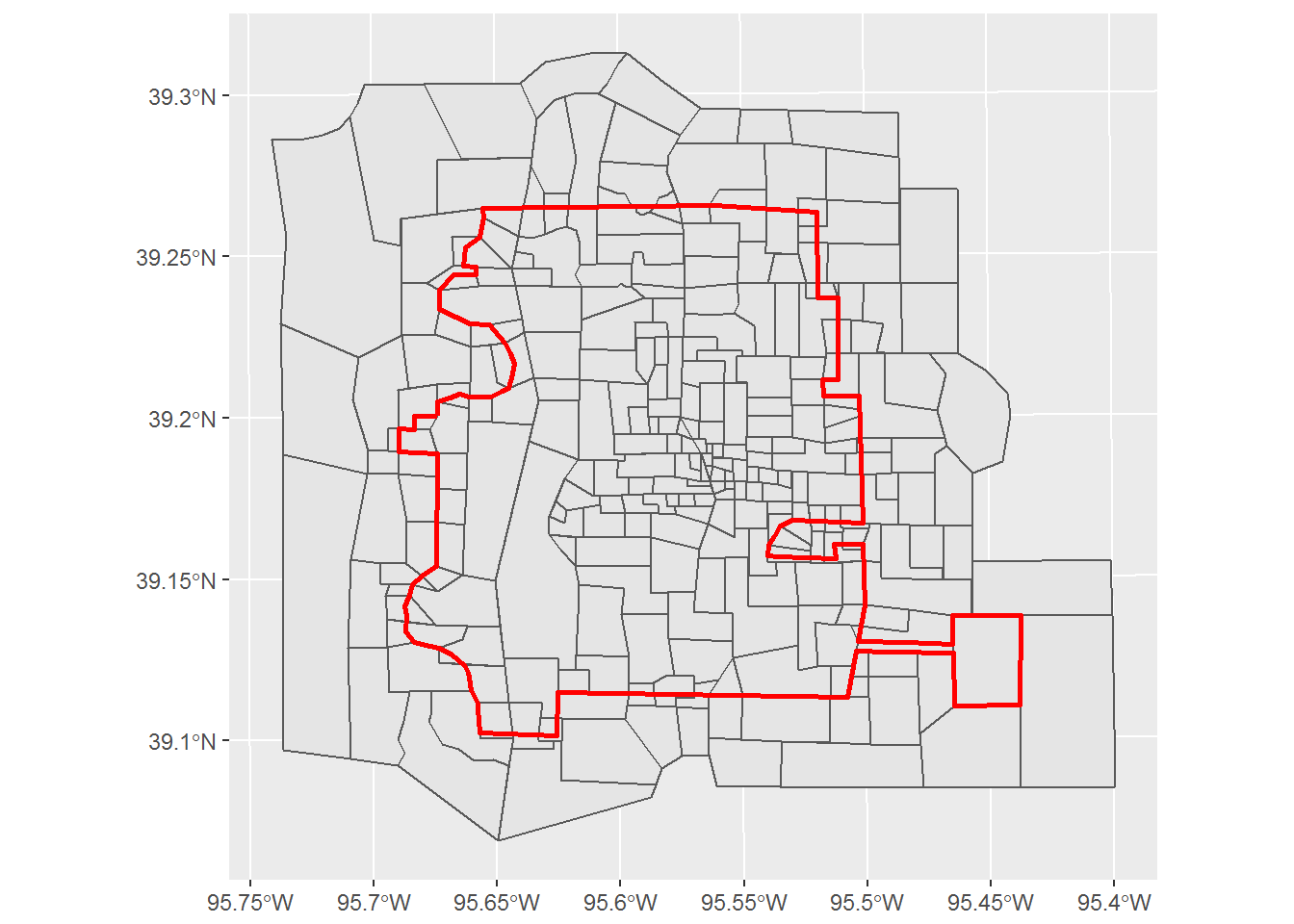From the vingette we find:

“The commands st_intersects, st_disjoint, st_touches, st_crosses, st_within, st_contains, st_overlaps, st_equals, st_covers, st_covered_by, st_equals_exact and st_is_within_distance return a sparse matrix with matching (TRUE) indexes, or a full logical matrix”

Thanks to Kyle Walker (here) we can use this matrix to subset those units that fall within certain locations relative to the city boundary.

### st_intersects

x1 <- st_intersects(geo_units, city_bnd)
x2 <- map_lgl(x1, function(x) {
if (length(x) == 1) {
return(TRUE)
} else {
return(FALSE)
}
})

ex1 <- geo_units[x2,]

ggplot() +
geom_sf(data = geo_units, lwd = .25, fill = NA) +
geom_sf(data = ex1, fill = "slategray2") +
geom_sf(data = city_bnd, fill = NA, color = "red", size = 1) +
ggtitle("sf geometrical operations", subtitle = "st_intersects")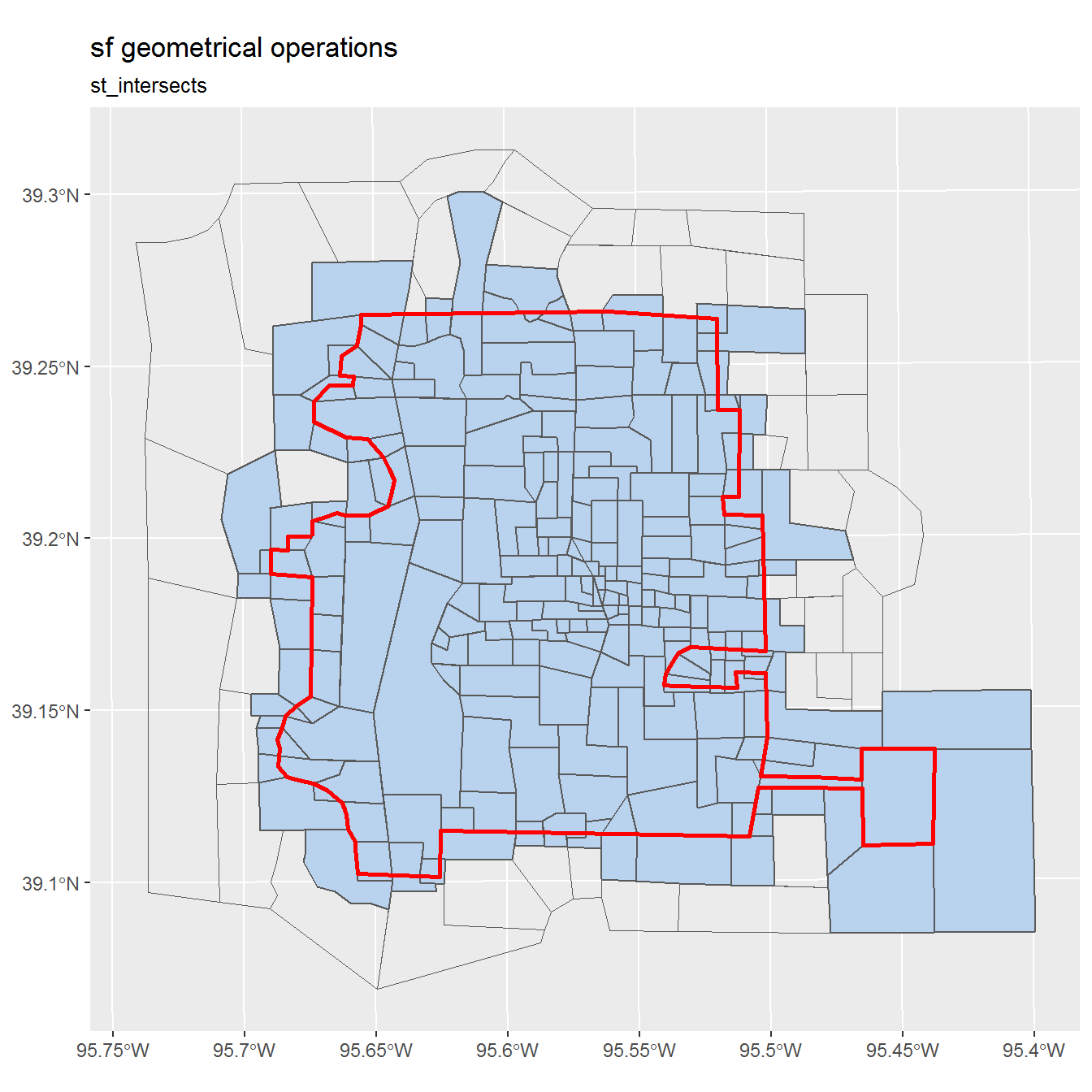We can see that intersecting includes when a polygon falls on the line of the city bounday too.

### st_disjoint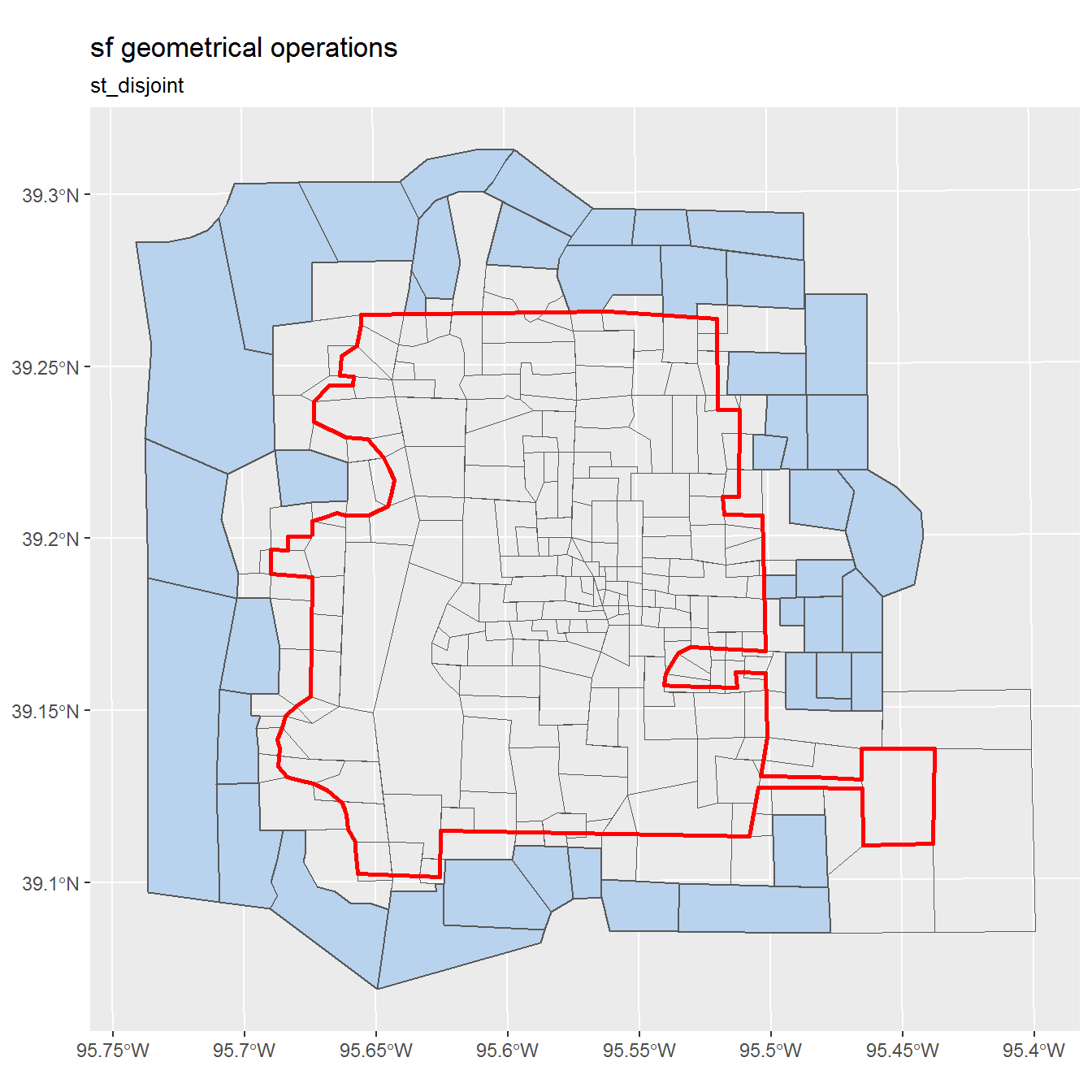st_disjoint appears to be the opposite of st_intersects, good to know.

### st_touches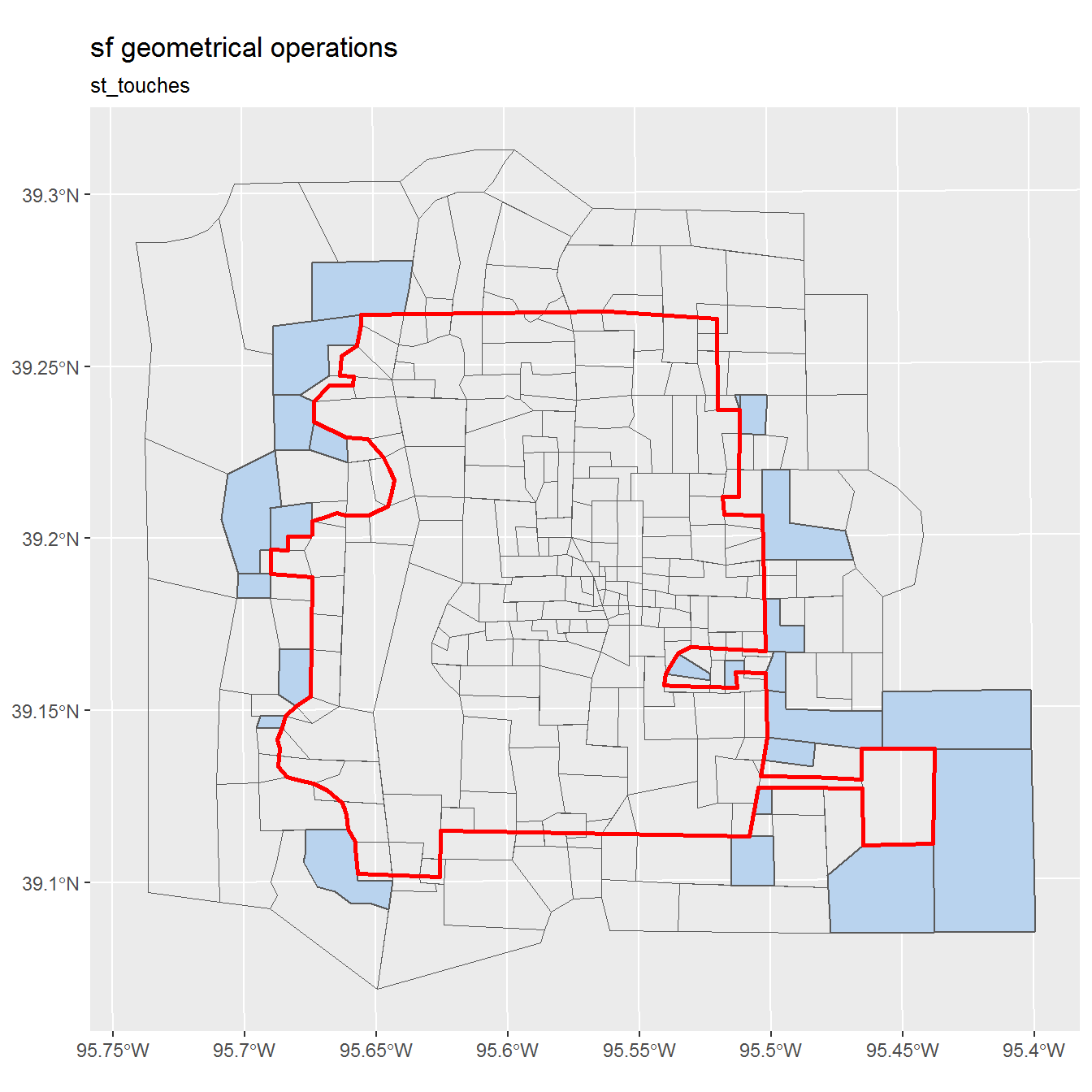Touches appears to mean on the outside of the city boundary only.

### st_crosses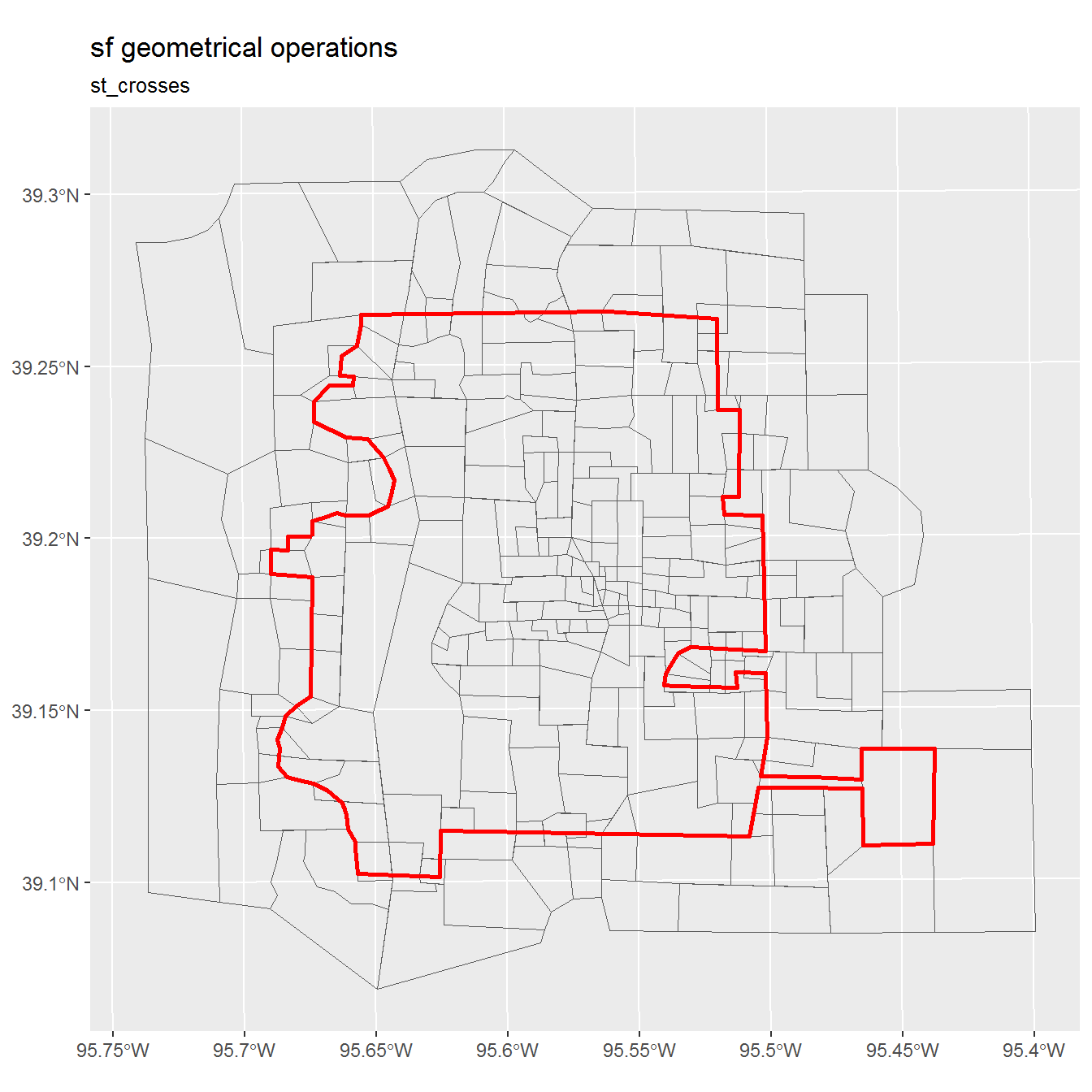Perhaps this shows that none of the unit polygons crosses the city boundary?

### st_within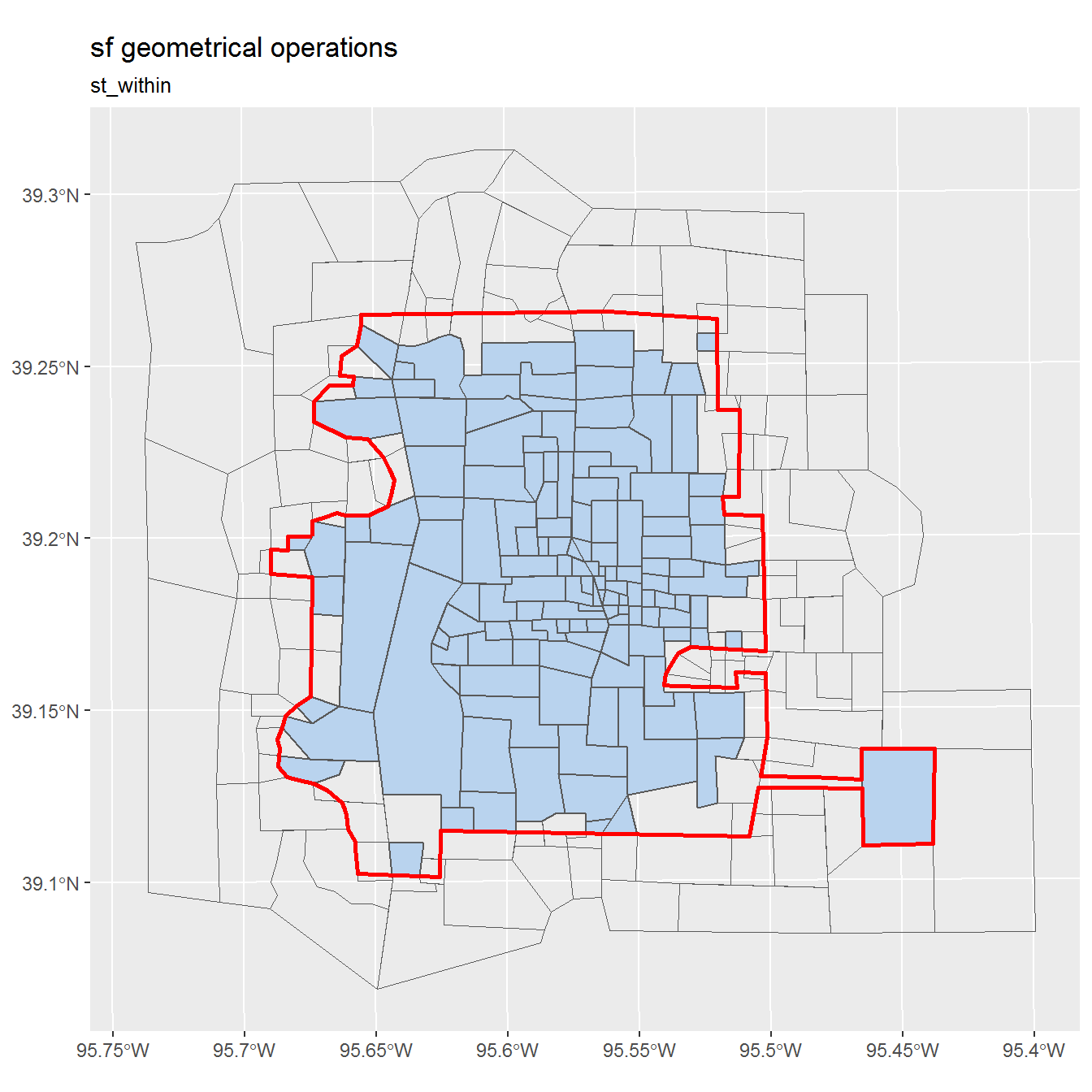### st_contains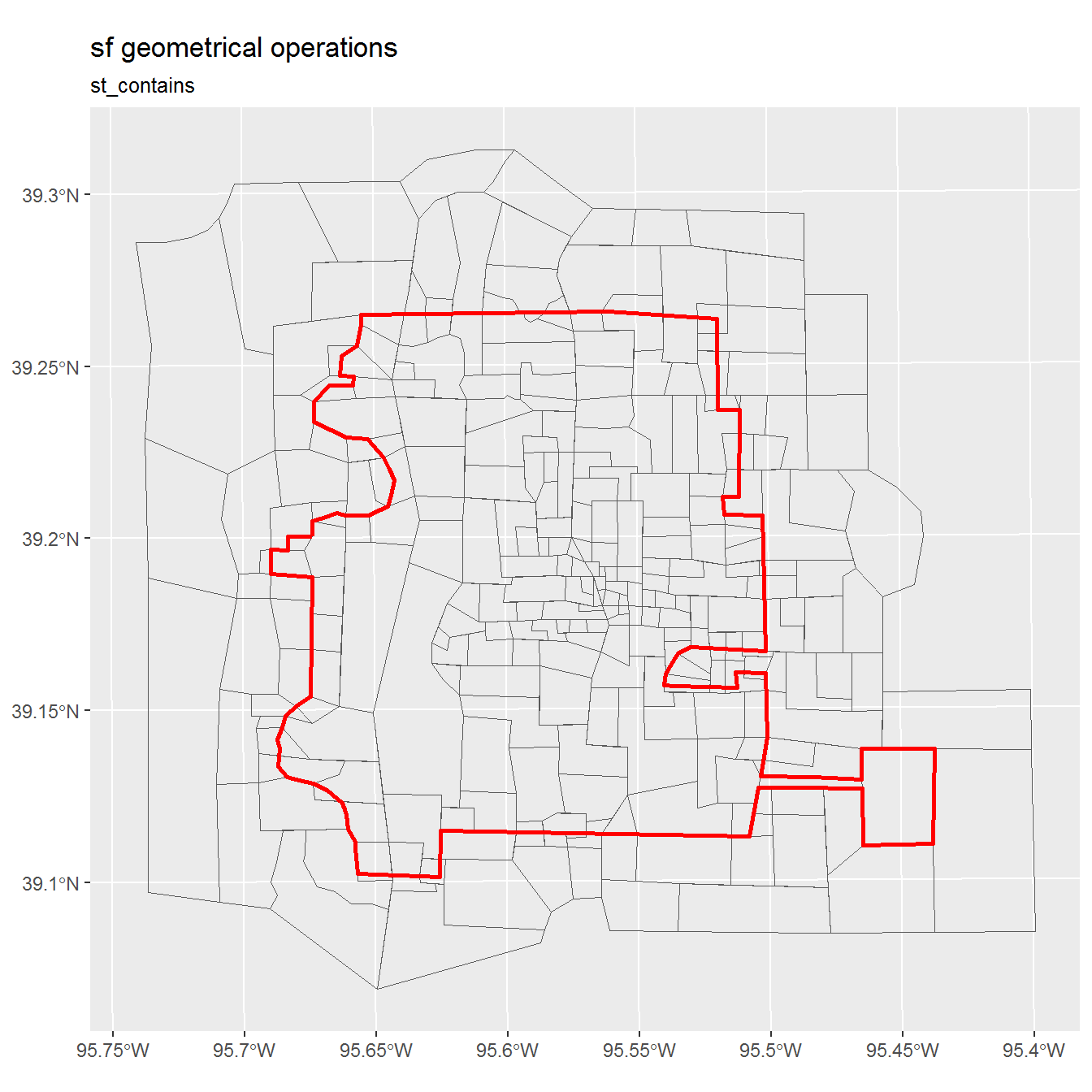### st_overlaps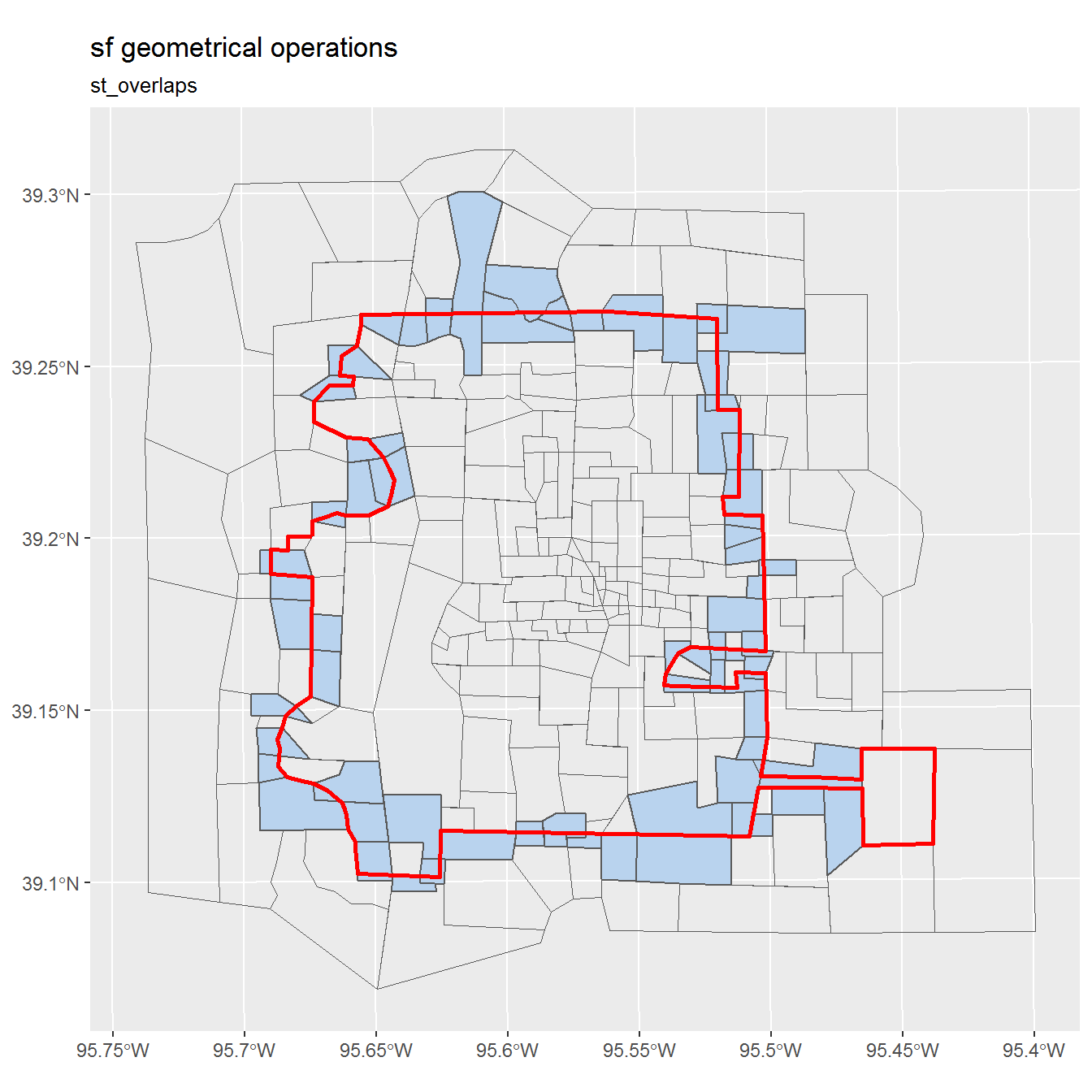### st_covered_by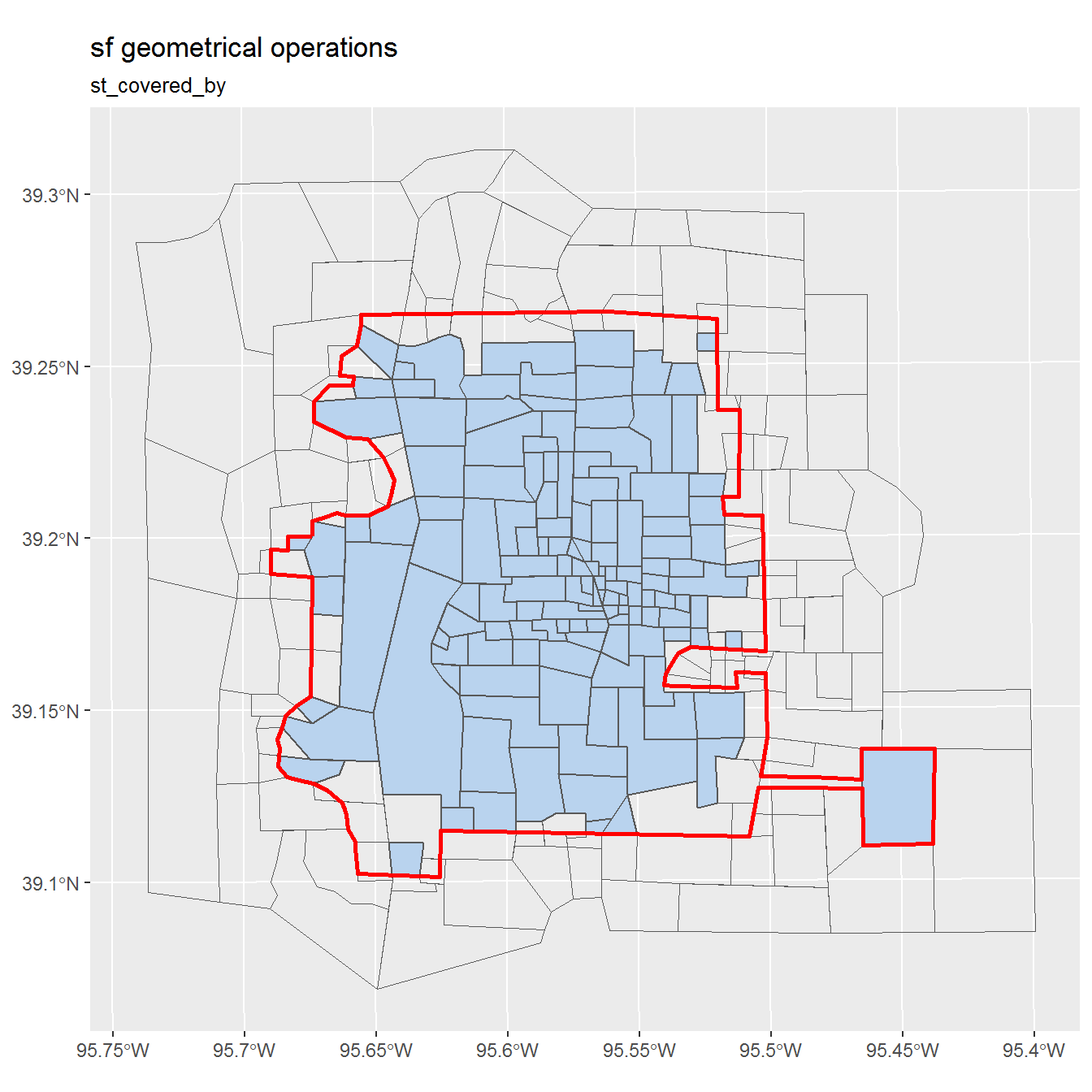</div>

</body>